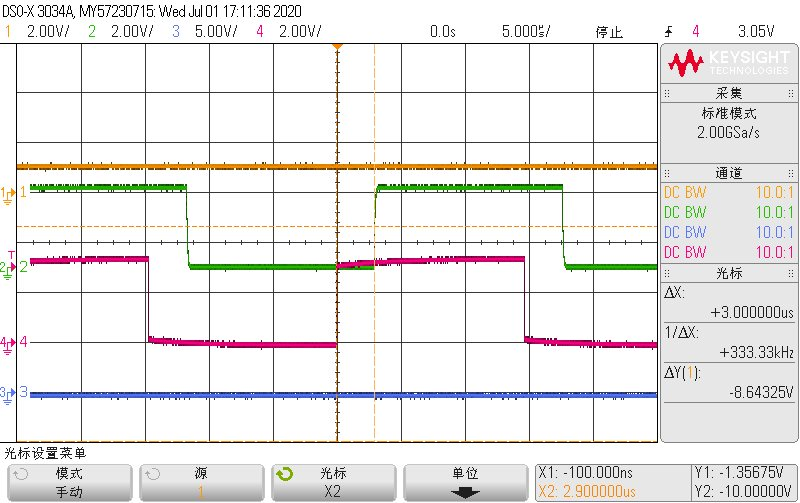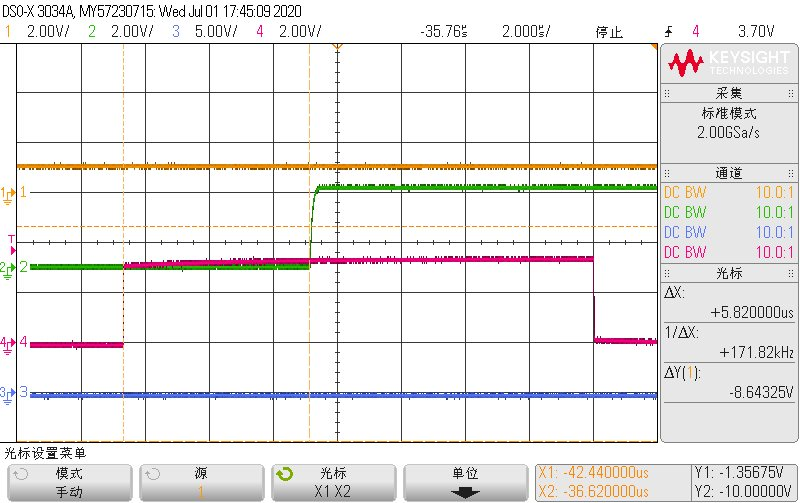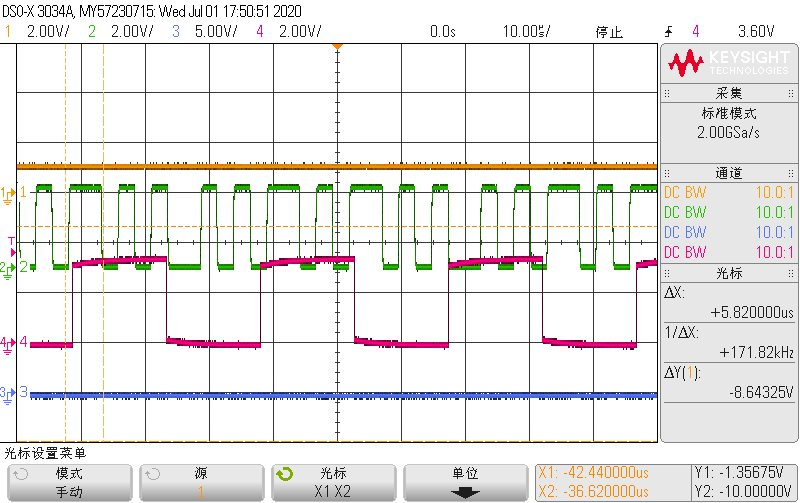If you have a related question, please click the "Ask a related question" button in the top right corner. The newly created question will be automatically linked to this question.

# TMS320F28020: how long does it take to convert 16 SOCs?

Part Number: TMS320F28020

Hi,

my customer met a strange phenomenon.

Their code is:

generate SOCA with EPWM4 count to 0 or PRD, every SOCA will trigger ADC conversion from SOC0-SOC15.

They also generate PWMISR at EPWM4 count to 0 or PRD, ISR happens every 15us.

We find that the result will only reflect the actual signal value 2 ISRs later, which is 30us.

Let's say at T=0us SOCA is triggered, at some time SOC15 start to convert. If all 16 SOCs can be converted within 15us, we should be able to read the correct result at PWMISR generated at T=15us. But now we are not able to do that, we have to wait another 15us to read the correct result in the next PWMISR.

For SOC1, we can read the correct result at PWMISR generated to T=15us.

The ADC setting code is as below:

```// Checkin \$Date: December 16, 2009   16:43:59 \$
//###########################################################################
//
//
// TITLE:   DSP2802x ADC Initialization & Support Functions.
//
//###########################################################################
// \$TI Release: 2802x C/C++ Header Files V1.26 \$
// \$Release Date: February 2, 2010 \$
//###########################################################################
//--------------------------------------------------------
// Product: Three Phase Vienna PFC
// Running on TMS320F2802x
// External clock is 20MHz, PLL * 4/2 , CPU-Clock 40 MHz
// Date: from 2011-10-9  , (Copyright) lvyougen
// Version:1.00
//--------------------------------------------------------

#include "DSP28x_Project.h"     // Headerfile Include File

//---------------------------------------------------------------------------
//---------------------------------------------------------------------------
// This function initializes ADC to a known state.
//
// NOTE: ADC INIT IS DIFFERENT ON 2802x DEVICES COMPARED TO OTHER 28X DEVICES
//
{
extern void DSP28x_usDelay(Uint32 Count);

// *IMPORTANT*
// The Device_cal function, which copies the ADC calibration values from TI reserved
// OTP into the ADCREFSEL and ADCOFFTRIM registers, occurs automatically in the
// Boot ROM. If the boot ROM code is bypassed during the debug process, the
// following function MUST be called for the ADC to function according
// to specification. The clocks to the ADC MUST be enabled before calling this
// function.
// See the device data manual and/or the ADC Reference

EALLOW;
(*Device_cal)();
EDIS;

// To powerup the ADC the ADCENCLK bit should be set first to enable
// clocks, followed by powering up the bandgap, reference circuitry, and ADC core.
// Before the first conversion is performed a 5ms delay must be observed
// after power up to give all analog circuits time to power up and settle

// Please note that for the delay function below to operate correctly the
// CPU_RATE define statement in the DSP2802x_Examples.h file must
// contain the correct CPU clock period in nanoseconds.
EALLOW;

EDIS;

EALLOW;

// and select to ADCINA5 for internal the temperature sensor

AdcRegs.ADCSOC0CTL.bit.ACQPS  = 6;	// SOCx Acquisition Prescale: Sample window is 7 cycles long (6 + 1 clock cycles).

StartSOC();	//enables SOCA pulse

EDIS;
}

{

EALLOW;

/* Configure ADC pins using AIO regs*/
// This specifies which of the possible AIO pins will be Analog input pins.
// Comment out other unwanted lines.

EDIS;
}

This function re-calibrates the ADC zero offset error by converting the VREFLO reference with
MUX select which connects VREFLO to A5 without sacrificing an external ADC pin. This
function calls two other functions:
*/
{
EALLOW;
AdcChanSelect(13);                                  //Select channel B5 for all SOC
AdcRegs.ADCOFFTRIM.bit.OFFTRIM = 80;                //Apply artificial offset (+80) to account for a negative offset that may reside in the ADC core
AdcRegs.ADCOFFTRIM.bit.OFFTRIM = 80 - AdcConvMean;  //Set offtrim register with new value (i.e remove artical offset (+80) and create a two's compliment of the offset error)
EDIS;
}

This function selects the ADC channel to convert by setting all SOC channel selects to a single channel.

* IMPORTANT * This function will overwrite previous SOC channel select settings. Recommend saving
the previous settings.
*/
{

to "ping-pong" between SOC0-7 and SOC8-15 and is referred to as "ping-pong" sampling.

* IMPORTANT * This function will overwrite previous ADC settings. Recommend saving previous settings.
*/
{
Uint16 index, SampleSize, Mean, ACQPS_Value;
Uint32 Sum;

index       = 0;            //initialize index to 0
SampleSize  = 256;          //set sample size to 256 (**NOTE: Sample size must be multiples of 2^x where is an integer >= 4)
Sum         = 0;            //set sum to 0
Mean        = 999;          //initialize mean to known value

//Set the ADC sample window to the desired value (Sample window = ACQPS + 1)
ACQPS_Value = 6;

//Enable ping-pong sampling

// Setup each SOC's ADCINT trigger source

while( index < SampleSize ){

index+=16;

} // end data collection

Mean = Sum / SampleSize;    //Calculate average ADC sample value

return Mean;                //return the average

//===========================================================================
// End of file.
//===========================================================================
```

• We've confirmed that SOC0-9 will not have such problem, means that the result we get 15us after AD conversion is triggered is correct.

but SOC10-15 will have such problem, means that we can only get the correct result 30us after AD conversion is triggered.

But in theory the whole 16 SOCs conversion should last shorter than 15us with 7 cycles sampling window.

• Howard,

-Tommy

• No, it's not reconfigured.

We did some more test by toggling a GPIO in EOC triggered ADCINT ISR.

If we configure end of conversion of SOC5 to trigger ADCINT, we can get the waveform below:

red rising edge - PWM COUNTER=0 which triggers SOCA to initiate all the AD conversion.

green rising edge- EOC of SOC5, we can see the delay is 3us, which is good.

For 6 SOCs conversion(from SOC0- SOC5), each SOC sampling window is 7 cycles, 13 conversion cycles, totally 20 cycles*6=120cycles* 0.025us= 3usIf we configure end of conversion of SOC7 to trigger ADCINT, we can get the waveform below:

the delay increased a lot to 5.8us, which doesn't make senseIf we configure end of conversion of SOC8 to trigger ADCINT, we can get the waveform below:

there will be multiple rising edge of the GREEN line(which means several EOC of SOC8) in one PWM cycle, which doesn't make sense at all.

What may cause the problem?• Howard Zou said:
No, it's not reconfigured.

• Sorry I don’t understand, we don’t need adcint to start soc . Why do we need to reconfigure it and how should we configure it?

• Because the AdcRegs.ADCINTSOCSELn registers are configured in `AdcConversion`(), which is used by `AdcOffsetSelfCal()`` in your code snippet. At the very least, you need to CLEAR the AdcRegs.ADCINTSOCSELn registers when configuring the ADC for EPWM-triggered operation.`

`Uint16 AdcConversion(``void``) `
`{ `
`    ``Uint16 index, SampleSize, Mean, ACQPS_Value; `
`    ``Uint32 Sum; `
`    ``index       = 0;            ``//initialize index to 0`` `
`    ``SampleSize  = 256;          ``//set sample size to 256 (**NOTE: Sample size must be multiples of 2^x where is an integer >= 4)`` `
`    ``Sum         = 0;            ``//set sum to 0`` `
`    ``Mean        = 999;          ``//initialize mean to known value`` `
`    ``//Set the ADC sample window to the desired value (Sample window = ACQPS + 1)`` `
`    ``ACQPS_Value = 6; `
`    ``AdcRegs.ADCSOC0CTL.bit.ACQPS  = ACQPS_Value; `
`    ``AdcRegs.ADCSOC1CTL.bit.ACQPS  = ACQPS_Value; `
`    ``AdcRegs.ADCSOC2CTL.bit.ACQPS  = ACQPS_Value; `
`    ``AdcRegs.ADCSOC3CTL.bit.ACQPS  = ACQPS_Value; `
`    ``AdcRegs.ADCSOC4CTL.bit.ACQPS  = ACQPS_Value; `
`    ``AdcRegs.ADCSOC5CTL.bit.ACQPS  = ACQPS_Value; `
`    ``AdcRegs.ADCSOC6CTL.bit.ACQPS  = ACQPS_Value; `
`    ``AdcRegs.ADCSOC7CTL.bit.ACQPS  = ACQPS_Value; `
`    ``AdcRegs.ADCSOC8CTL.bit.ACQPS  = ACQPS_Value; `
`    ``AdcRegs.ADCSOC9CTL.bit.ACQPS  = ACQPS_Value; `
`    ``AdcRegs.ADCSOC10CTL.bit.ACQPS = ACQPS_Value; `
`    ``AdcRegs.ADCSOC11CTL.bit.ACQPS = ACQPS_Value; `
`    ``AdcRegs.ADCSOC12CTL.bit.ACQPS = ACQPS_Value; `
`    ``AdcRegs.ADCSOC13CTL.bit.ACQPS = ACQPS_Value; `
`    ``AdcRegs.ADCSOC14CTL.bit.ACQPS = ACQPS_Value; `
`    ``AdcRegs.ADCSOC15CTL.bit.ACQPS = ACQPS_Value; `
`    ``//Enable ping-pong sampling`` `
`    ``// Enabled ADCINT1 and ADCINT2`` `
`    ``AdcRegs.INTSEL1N2.bit.INT1E = 1; `
`    ``AdcRegs.INTSEL1N2.bit.INT2E = 1; `
`    ``// Disable continuous sampling for ADCINT1 and ADCINT2`` `
`    ``AdcRegs.INTSEL1N2.bit.INT1CONT = 0; `
`    ``AdcRegs.INTSEL1N2.bit.INT2CONT = 0; `
`    ``AdcRegs.ADCCTL1.bit.INTPULSEPOS = 1;    ``//ADCINTs trigger at end of conversion`` `
`    ``// Setup ADCINT1 and ADCINT2 trigger source`` `
`    ``AdcRegs.INTSEL1N2.bit.INT1SEL = 6;      ``//EOC6 triggers ADCINT1`` `
`    ``AdcRegs.INTSEL1N2.bit.INT2SEL = 14;     ``//EOC14 triggers ADCINT2`` `
`    ``// Setup each SOC's ADCINT trigger source`` `
`    ``AdcRegs.ADCINTSOCSEL1.bit.SOC0  = 2;    ``//ADCINT2 starts SOC0-7`` `
`    ``AdcRegs.ADCINTSOCSEL1.bit.SOC1  = 2; `
`    ``AdcRegs.ADCINTSOCSEL1.bit.SOC2  = 2; `
`    ``AdcRegs.ADCINTSOCSEL1.bit.SOC3  = 2; `
`    ``AdcRegs.ADCINTSOCSEL1.bit.SOC4  = 2; `
`    ``AdcRegs.ADCINTSOCSEL1.bit.SOC5  = 2; `
`    ``AdcRegs.ADCINTSOCSEL1.bit.SOC6  = 2; `
`    ``AdcRegs.ADCINTSOCSEL1.bit.SOC7  = 2; `
`    ``AdcRegs.ADCINTSOCSEL2.bit.SOC8  = 1;    ``//ADCINT1 starts SOC8-15`` `
`    ``AdcRegs.ADCINTSOCSEL2.bit.SOC9  = 1; `
`    ``AdcRegs.ADCINTSOCSEL2.bit.SOC10 = 1; `
`    ``AdcRegs.ADCINTSOCSEL2.bit.SOC11 = 1; `
`    ``AdcRegs.ADCINTSOCSEL2.bit.SOC12 = 1; `
`    ``AdcRegs.ADCINTSOCSEL2.bit.SOC13 = 1; `
`    ``AdcRegs.ADCINTSOCSEL2.bit.SOC14 = 1; `
`    ``AdcRegs.ADCINTSOCSEL2.bit.SOC15 = 1; `
• Thank you.

You are correct.

I've got another question: